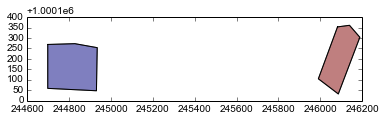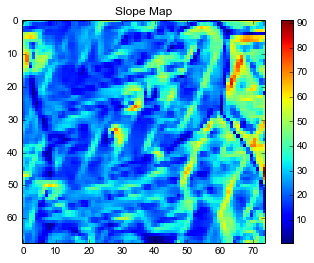# Integrating with GeoPandas and Numpy¶

rasterstats has mainly operated on file-based vector and raster data sources. As of version 0.4, we've added the ability to work with in-memory representations of vector and raster data:

• GeoPandas provides a GeoDataFrame for vector data
• Numpy provide ndarrays which can be used as a raster data source (with some additional metadata for georeferencing)

In this example you'll learn how to load vectors into GeoDataFrames and rasters into ndarrays and inspect the results. Then we'll run zonal statistics on the two datasets, bring it back into a GeoDataFrames and write the results to a GeoJSON file.

### Vector Data via GeoPandas¶

Let's load up some example vector data into a GeoDataFrame. We can see the shapes plotted as a map

In :
import geopandas as gpd
geodf = gpd.GeoDataFrame.from_file('../tests/data/polygons.shp')
geodf.plot()

Out:
<matplotlib.axes.AxesSubplot at 0x7f2a25414090>... or view it as a table

In :
geodf

Out:
geometry id
0 POLYGON ((244697.4517952438 1000369.230757494,... 1
1 POLYGON ((246082.2236020251 1000453.156321541,... 2

### Raster Data via Numpy¶

We can use the rasterio package to bring GDAL-supported data formats into our python session as numpy arrays. Note that we need to keep track of the georeferencing explicitly by storing the transform tuple.

In :
import rasterio
with rasterio.open("../tests/data/slope.tif") as src:
transform = src.meta['transform']

print (transform)
array

[244300.61494985913, 25.52514657450613, 0.0, 1000868.7876863468, 0.0, -25.52514657450613]

Out:
array([[-9999., -9999., -9999., ..., -9999., -9999., -9999.],
[-9999.,     0.,     0., ...,     0.,     0., -9999.],
[-9999.,     0.,     0., ...,     0.,     0., -9999.],
...,
[-9999.,     0.,     0., ...,     0.,     0., -9999.],
[-9999.,     0.,     0., ...,     0.,     0., -9999.],
[-9999., -9999., -9999., ..., -9999., -9999., -9999.]], dtype=float32)

Because it's an ndarray, we have the entire numpy and scipy world available to us to work on the data.

In :
array_chop = array[5:-5,5:-5]  # chop off the outer 5 pixels


With a few lines of matplotlib glue, we can plot a map of the data.

In :
import matplotlib.pyplot as plt
plt.imshow(array_chop, interpolation='nearest')
plt.colorbar()
plt.title('Slope Map')
plt.show()### Running zonal statistics on GeoDataFrames and ndarrays¶

Now that we have our vectors and raster data loaded into the python session, we can work with zonal_stats directly rather than serializing them to disk.

In :
from rasterstats import zonal_stats
zonal_stats(geodf, array)

---------------------------------------------------------------------------
RasterStatsError                          Traceback (most recent call last)
<ipython-input-11-c44947387cef> in <module>()
1 from rasterstats import zonal_stats
----> 2 zonal_stats(geodf, array)

/home/mperry/src/python-raster-stats/src/rasterstats/main.pyc in zonal_stats(vectors, raster, layer_num, band, nodata_value, global_src_extent, categorical, stats, copy_properties, all_touched, transform)
58         # must have transform arg
59         if not transform:
---> 60             raise RasterStatsError("Must provide the 'transform' kwarg when "\
61                 "using ndarrays as src raster")
62         rgt = transform

RasterStatsError: Must provide the 'transform' kwarg when using ndarrays as src raster

What happened? Because the ndarrays are not geo-aware (they are just in array coordinates) we need to specify the transform tuple to specify exactly how the cells align with our spatial reference system.

The tuple contains 6 elements which correspond to:

1. top left easting or X coordinate
2. West-East pixel resolution
3. rotation (typically 0 if image is "north up")
4. top left northing or Y coordinate
5. rotation, (typically 0 if image is "north up")
6. North-South pixel resolution (typically -1 * W-E resolution)

You can construct this by hand if necessary but it's handy to use the coordinates from the source dataset, provided the dimensions of the array have not changed.

In :
transform

Out:
[244300.61494985913,
25.52514657450613,
0.0,
1000868.7876863468,
0.0,
-25.52514657450613]

With the array and the transform information, we can run zonal_stats without complaint.

In :
stats = zonal_stats(geodf, array, transform=transform)
stats

Out:
[{'__fid__': 0,
'count': 75,
'max': 22.273418426513672,
'mean': 14.660084635416666,
'min': 6.575114727020264},
{'__fid__': 1,
'count': 50,
'max': 82.69043731689453,
'mean': 56.6057470703125,
'min': 16.940950393676758}]

### Back to GeoDataFrames¶

The "list of dicts" representation of zonal statistics is helpful but we will often want to join these attributes back to the original GeoDataFrame in order to continue working with them. For example, we may want to do some additional calculations and write it out to a GeoJSON file.

Luckily (geo)pandas has some very intuitive constructors and methods that make this simple:

In :
import pandas as pd

new_geodf = geodf.join(pd.DataFrame(stats))
new_geodf

Out:
geometry id __fid__ count max mean min
0 POLYGON ((244697.4517952438 1000369.230757494,... 1 0 75 22.273418 14.660085 6.575115
1 POLYGON ((246082.2236020251 1000453.156321541,... 2 1 50 82.690437 56.605747 16.940950

And to write it out to a vector file like GeoJSON

This currently does not work because the geodf.join method returns as standard DataFrame, not a GeoDataFrame! This bug should be fixed shortly...

In :
## Hack around it for now
new_geodf.__class__ = gpd.GeoDataFrame
new_geodf.crs = {}
new_geodf.set_geometry('geometry')
## /hack

! rm /tmp/polygons_with_slope.geojson
new_geodf.to_file('/tmp/polygons_with_slope.geojson', driver="GeoJSON")

# Confirm that everything works according to OGR
! ogrinfo -ro -al /tmp/polygons_with_slope.geojson

INFO: Open of /tmp/polygons_with_slope.geojson'
using driver GeoJSON' successful.

Layer name: OGRGeoJSON
Geometry: Polygon
Feature Count: 2
Extent: (244697.451795, 1000132.713259) - (246189.037956, 1000460.785918)
Layer SRS WKT:
GEOGCS["WGS 84",
DATUM["WGS_1984",
SPHEROID["WGS 84",6378137,298.257223563,
AUTHORITY["EPSG","7030"]],
AUTHORITY["EPSG","6326"]],
PRIMEM["Greenwich",0,
AUTHORITY["EPSG","8901"]],
UNIT["degree",0.0174532925199433,
AUTHORITY["EPSG","9122"]],
AUTHORITY["EPSG","4326"]]
FID Column = id
id: Integer (0.0)
__fid__: Integer (0.0)
count: Integer (0.0)
max: Real (0.0)
mean: Real (0.0)
min: Real (0.0)
OGRFeature(OGRGeoJSON):1
id (Integer) = 1
__fid__ (Integer) = 0
count (Integer) = 75
max (Real) = 22.2734184265137
mean (Real) = 14.6600846354167
min (Real) = 6.57511472702026
POLYGON ((244697.451795243832748 1000369.230757493642159,244827.154939680622192 1000373.045555859454907,244933.969293922709767 1000353.971564030507579,244933.969293922709767 1000353.971564030507579,244930.154495556926122 1000147.97245227789972,244697.451795243832748 1000159.41684737522155,244697.451795243832748 1000369.230757493642159))

OGRFeature(OGRGeoJSON):2
id (Integer) = 2
__fid__ (Integer) = 1
count (Integer) = 50
max (Real) = 82.6904373168945
mean (Real) = 56.6057470703125
min (Real) = 16.9409503936768
POLYGON ((246082.223602025071159 1000453.156321540940553,246139.445577511884039 1000460.78591827256605,246189.03795626712963 1000403.563942785724066,246189.03795626712963 1000403.563942785724066,246086.038400390854804 1000132.71325881476514,245990.668441246147268 1000205.194427764741704,246082.223602025071159 1000453.156321540940553))